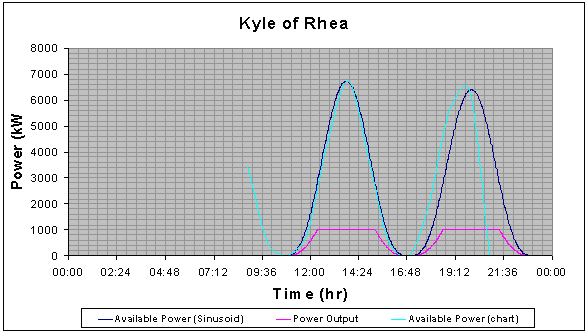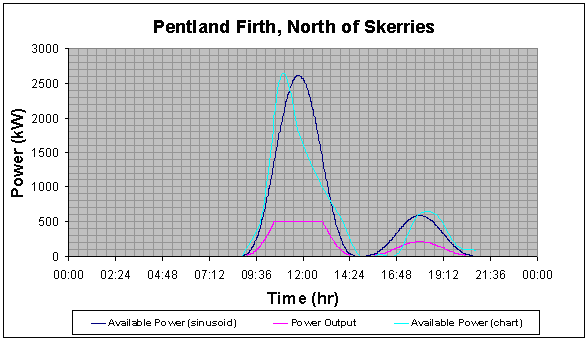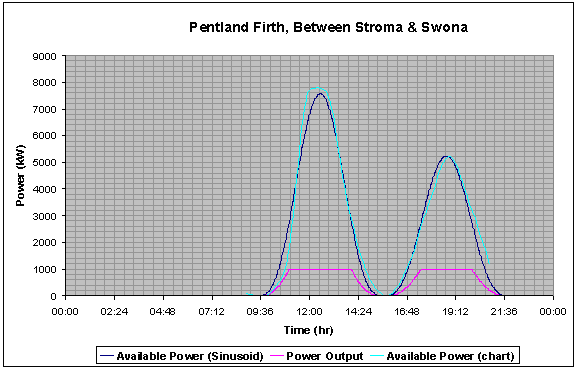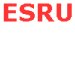Calculations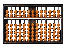Sample Results

A sample of the Microsoft Excel Worksheet for each location is as shown below; it generates the sinusoidal model, calculates the energy outputs as well as the error estimation for a given set of basic tidal data and turbine parameters.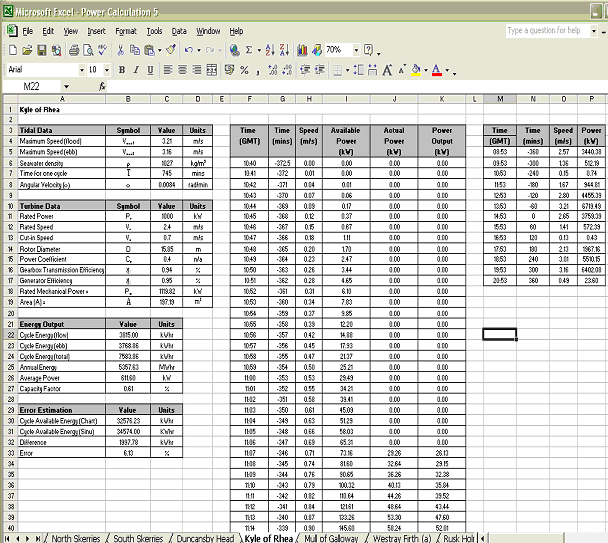Samples of graphs generated for each location are as below. These graphs show the power output profile based on the sinusoidal model. They also show how well the sinusoidal model matches to the chart data.